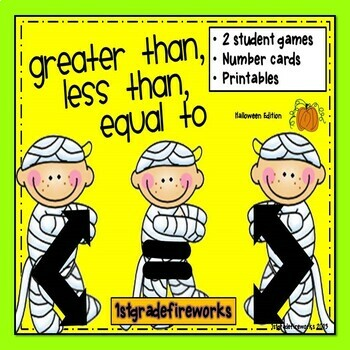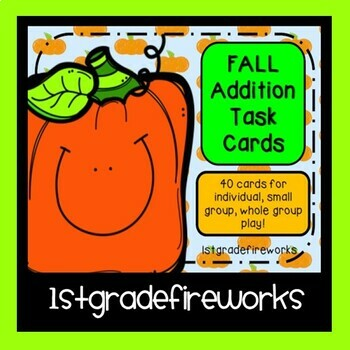# Howlin' Halloween MATH Mega-BUNDLEK - 3rd
Subjects
Standards
Resource Type
Formats Included
• Zip
\$9.99
Bundle
List Price:
\$10.98
You Save:
\$0.99
\$9.99
Bundle
List Price:
\$10.98
You Save:
\$0.99

### Description

Huge Math Mega-Bundle for Halloween!

Includes:

• Greater Than, Less Than Halloween Edition

( 2 games for students to play together!)

• Halloween Math Facts - True or False?

(Pocket Chart Sorting Game)

• Spooktacular Math Dominoes

( 116 Domino cards for playing)

• Candy Corn Fact Family FREEBIE

Total Pages
N/A
Teaching Duration
N/A
Report this Resource to TpT
Reported resources will be reviewed by our team. Report this resource to let us know if this resource violates TpT’s content guidelines.

### Standards

to see state-specific standards (only available in the US).
Understand the meaning of the equal sign, and determine if equations involving addition and subtraction are true or false. For example, which of the following equations are true and which are false? 6 = 6, 7 = 8 - 1, 5 + 2 = 2 + 5, 4 + 1 = 5 + 2.
Add and subtract within 20, demonstrating fluency for addition and subtraction within 10. Use strategies such as counting on; making ten (e.g., 8 + 6 = 8 + 2 + 4 = 10 + 4 = 14); decomposing a number leading to a ten (e.g., 13 - 4 = 13 - 3 - 1 = 10 - 1 = 9); using the relationship between addition and subtraction (e.g., knowing that 8 + 4 = 12, one knows 12 - 8 = 4); and creating equivalent but easier or known sums (e.g., adding 6 + 7 by creating the known equivalent 6 + 6 + 1 = 12 + 1 = 13).
Apply properties of operations as strategies to add and subtract. If 8 + 3 = 11 is known, then 3 + 8 = 11 is also known. (Commutative property of addition.) To add 2 + 6 + 4, the second two numbers can be added to make a ten, so 2 + 6 + 4 = 2 + 10 = 12. (Associative property of addition.)